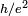# Ohm

The ohm, abbreviated Ω(Omega), is the SI unit of electrical resistance. It is the resistance which will allow a current of one ampere across a potential drop of one volt.

The ohm is named for Georg Ohm (1789 - 1854), an early investigator of electricity, who determined the relation between current, potential, and resistance, now called Ohm's Law.

The ohm is a derived unit in the SI, equal to 1 V/A; or in terms of SI basic units:$\Omega = m^2 \cdot kg \cdot s^{-3} \cdot A^{-2} = \frac{m^2 \cdot kg}{s^{3} \cdot A^{2}}$.

## Other definitions

The "international ohm" was defined in 1893 (at the International Electrical Conference) as the resistance of a column of mercury of constant cross section at the temperature of melting ice, 106.3 centimeters long and with a mass of 14.4521 grams (which gave a cross-section of 1 square millimeter).

In 1990, the CIPM recommended that a conventional value of 25812.807 Ω be used for the von Klitzing constant$h/e^2$ (where h is Planck's constant and e is the elementary charge), which makes calibration easy using the quantum Hall effect. This is technically not a redefinition, but allows for increased precision in measurement.

## Sources

• Ohm. Sizes.com (2005-02-14). Retrieved on 2007-06-23.
• International ohm. Sizes.com (2007-06-03). Retrieved on 2007-06-23.Some content on this page may previously have appeared on Citizendium.